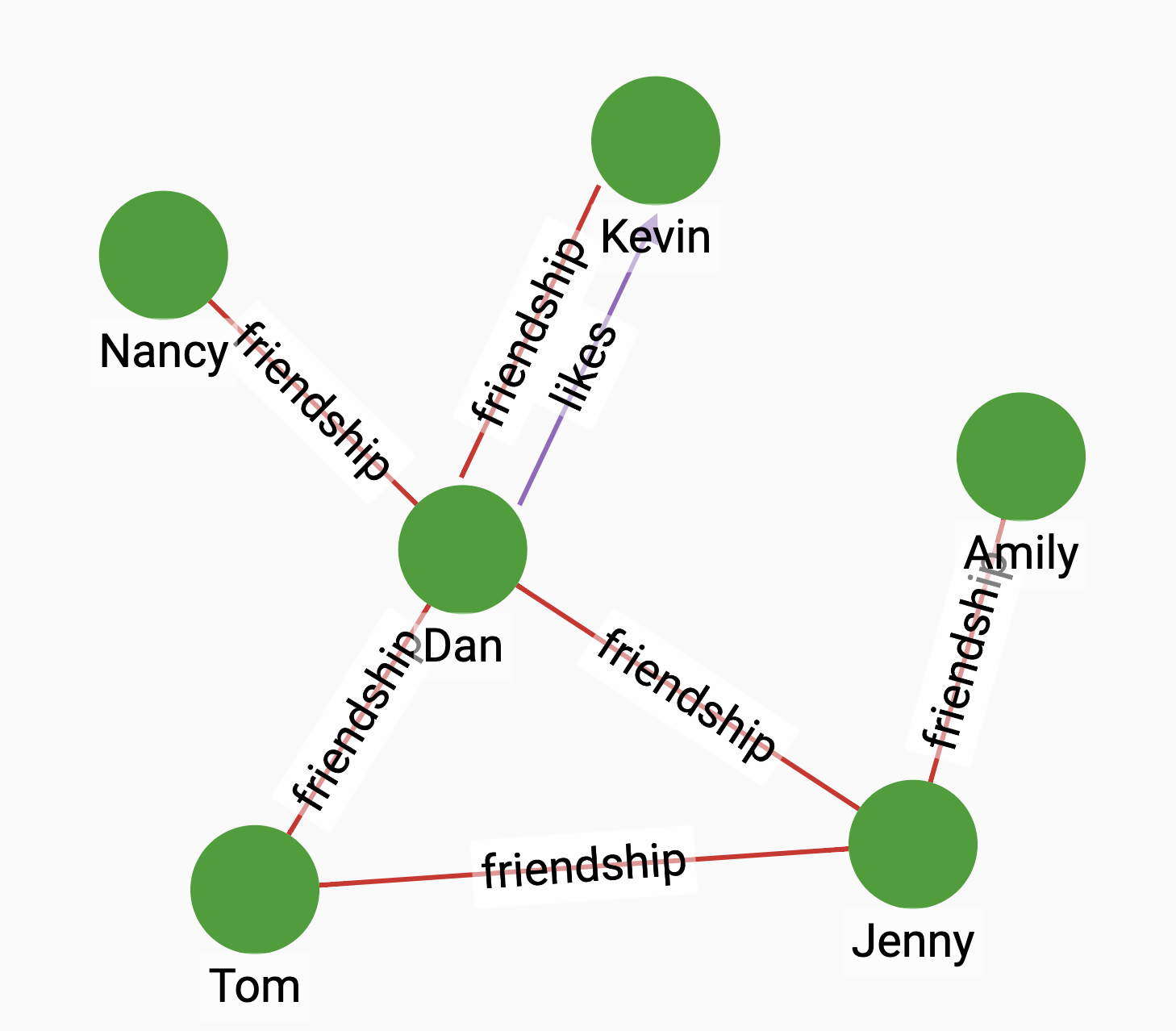# Resource Allocation

Resource Allocation is used to compute the closeness of nodes based on their shared neighbors. It is computed by the following formula:

${RA(x,y)=\sum _{u\in N(x)\cap N(y)}{\frac {1} {|N(u)|}}}$

Where ${N(u)}$ is the set of nodes adjacent to u.

## Specification

``CREATE QUERY tg_resource_allocation(VERTEX a, VERTEX b, SET<STRING> e_type)``

### Time complexity

The algorithm has a time complexity of $O(D1 + D2)$, where D1 and D2 are the degrees of the two vertices.

## Parameters

Name Description Data type

`a`

A vertex.

`VERTEX`

`b`

A vertex.

`VERTEX`

`e_type`

Edge types to traverse.

`SET<STRING>`

## Example

Suppose we have the following graph:Since Dan and Jenny has one shared neighbor `Tom`, who has two neighbors, running the algorithm between Dan and Jenny with friendship edges would give us a result of 0.5.

• Query

• Result

``RUN QUERY resource_allocation (("Jenny", "person"), ("Dan", "person"), ["friendship"])``
``````{
"error": false,
"message": "",
"version": {
"schema": 1,
"edition": "enterprise",
"api": "v2"
},
"results": [{
"@@closeness": 0.5
}]
}``````